# WLAN Channel Models

This example demonstrates passing WLAN S1G, VHT, HT, and non-HT format waveforms through appropriate fading channel models. When simulating a WLAN communications link, viable options for channel modeling include the TGah,TGn and TGac models from WLAN Toolbox™ and the additive white Gaussian noise (AWGN) and 802.11g models from Communications Toolbox™. In this example, it is sufficient to set the channel model sampling frequency to match the channel bandwidth because no front-end filtering is applied to the signal and the oversampling rate is 1.

In each section of this example, you:

• Create a waveform.

• Use a spectrum analyzer to display the waveform before and after it passes through the noisy fading channel.

### Pass S1G Waveform Through TGah SISO Channel

Create a bit stream to use when generating the WLAN S1G format waveform.

```bits = randi([0 1],1000,1); ```

Create an S1G configuration object, and then generate a 2 MHz S1G waveform. Calculate the signal power.

```s1g = wlanS1GConfig; preChS1G = wlanWaveformGenerator(bits,s1g); ```

Pass the signal through a TGah SISO channel with AWGN noise (SNR=10 dB) and a receiver with a 9 dB noise figure. Recall that the channel model sampling frequency is equal to the bandwidth in this example. Set property values by using name-value pairs.

Create a TGah channel object. Set the channel model sampling frequency and channel bandwidth, enable path loss and shadowing, and use the Model-D delay profile.

```cbw = s1g.ChannelBandwidth; fs = 2e6; % Channel model sampling frequency equals the channel bandwidth tgahChan = wlanTGahChannel('SampleRate',fs,'ChannelBandwidth',cbw, ... 'LargeScaleFadingEffect','Pathloss and shadowing', ... 'DelayProfile','Model-D'); ```

Create an `AWGN Channel` object with SNR = 10 dB. Determine the signal power in Watts, accounting for the TGah large scale fading pathloss.

```preChSigPwr_dB = 20*log10(mean(abs(preChS1G))); sigPwr = 10^((preChSigPwr_dB-tgahChan.info.Pathloss)/10); chNoise = comm.AWGNChannel('NoiseMethod','Signal to noise ratio (SNR)',... 'SNR',10,'SignalPower', sigPwr); ```

Pass the S1G waveform through a SISO TGah channel and add the AWGN channel noise.

```postChS1G = chNoise(tgahChan(preChS1G)); ```

Create another `AWGN Channel` object to add receiver noise.

```rxNoise = comm.AWGNChannel('NoiseMethod','Variance', ... 'VarianceSource','Input port'); ```

Pass the S1G waveform through the receiver. Choose an appropriate noise variance, nVar, to set the receiver noise level. Here, the receiver noise level is based on the noise variance for a receiver with a 9 dB noise figure. `nVar` = kTBF, where k is Boltzmann's constant, T is the ambient temperature of 290 K, B is the bandwidth, and F is the receiver noise figure.

```nVar = 10^((-228.6 + 10*log10(290) + 10*log10(fs) + 9)/10); rxS1G = rxNoise(postChS1G,nVar); ```

Display a spectrum analyzer with before-channel and after-channel waveforms. Use `SpectralAverages` = 10 to reduce noise in the plotted signals.

```title = '2 MHz S1G Waveform Before and After TGah Channel'; saScope = dsp.SpectrumAnalyzer('SampleRate',fs,'ShowLegend',true,... 'AveragingMethod','Exponential','ForgettingFactor',0.99,'Title',title,... 'ChannelNames',{'Before','After'}); saScope([preChS1G,rxS1G]) ```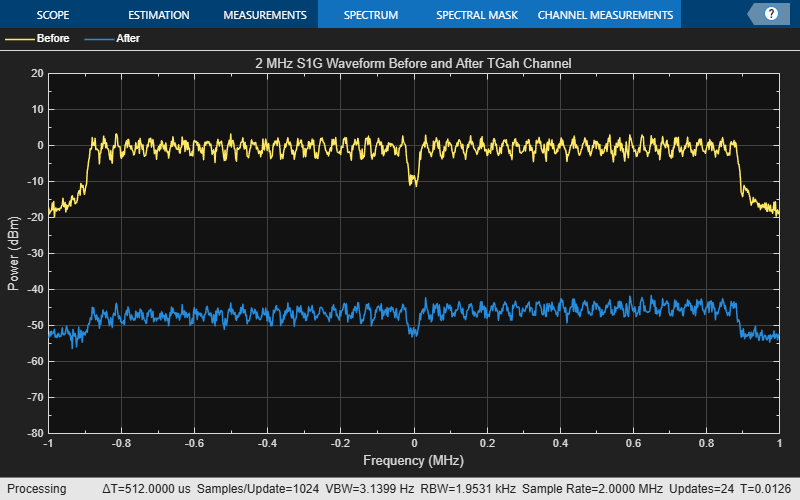Path loss accounts for the roughly 50 dB of separation between the waveform before and after it passes through the TGah channel. The path loss results from the default transmitter-to-receiver distance of 3 meters, and from shadowing effects. The signal level variation shows the frequency selectivity of the delay profile across the frequency spectrum.

### Pass VHT Waveform Through TGac SISO Channel

Create a bit stream to use when generating the WLAN VHT format waveform.

```bits = randi([0 1],1000,1); ```

Create a VHT configuration object, and generate an 80 MHz VHT waveform. Calculate the signal power.

```vht = wlanVHTConfig; preChVHT = wlanWaveformGenerator(bits,vht); ```

Pass the signal through a TGac SISO channel with AWGN noise (SNR=10 dB) and a receiver with a 9 dB noise figure. Recall that the channel model sampling frequency is equal to the bandwidth in this example. Set parameters using `Name,Value` pairs.

Create a TGac channel object. Set the channel model sampling frequency and channel bandwidth, enable path loss and shadowing, and use the Model-D delay profile.

```cbw = vht.ChannelBandwidth; fs = 80e6; % Channel model sampling frequency equals the channel bandwidth tgacChan = wlanTGacChannel('SampleRate',fs,'ChannelBandwidth',cbw, ... 'LargeScaleFadingEffect','Pathloss and shadowing', ... 'DelayProfile','Model-D'); ```

Create an `AWGN Channel` object with SNR = 10 dB. Determine the signal power in Watts, accounting for the TGac large scale fading pathloss.

```preChSigPwr_dB = 20*log10(mean(abs(preChVHT))); sigPwr = 10^((preChSigPwr_dB-tgacChan.info.Pathloss)/10); chNoise = comm.AWGNChannel('NoiseMethod','Signal to noise ratio (SNR)',... 'SNR',10,'SignalPower', sigPwr); ```

Pass the VHT waveform through a SISO TGac channel and add the AWGN channel noise.

```postChVHT = chNoise(tgacChan(preChVHT)); ```

Create another `AWGN Channel` object to add receiver noise.

```rxNoise = comm.AWGNChannel('NoiseMethod','Variance', ... 'VarianceSource','Input port'); ```

Pass the VHT waveform through the receiver. Choose an appropriate noise variance, nVar, to set the receiver noise level. Here, the receiver noise level is based on the noise variance for a receiver with a 9 dB noise figure. `nVar` = kTBF, where k is Boltzmann's constant, T is the ambient temperature of 290 K, B is the bandwidth, and F is the receiver noise figure.

```nVar = 10^((-228.6 + 10*log10(290) + 10*log10(fs) + 9)/10); rxVHT = rxNoise(postChVHT,nVar); ```

Display a spectrum analyzer with before-channel and after-channel waveforms. Use `SpectralAverages` = 10 to reduce noise in the plotted signals.

```title = '80 MHz VHT Waveform Before and After TGac Channel'; saScope = dsp.SpectrumAnalyzer('SampleRate',fs,'ShowLegend',true,... 'AveragingMethod','Exponential','ForgettingFactor',0.99,'Title',title,... 'ChannelNames',{'Before','After'}); saScope([preChVHT,rxVHT]) ```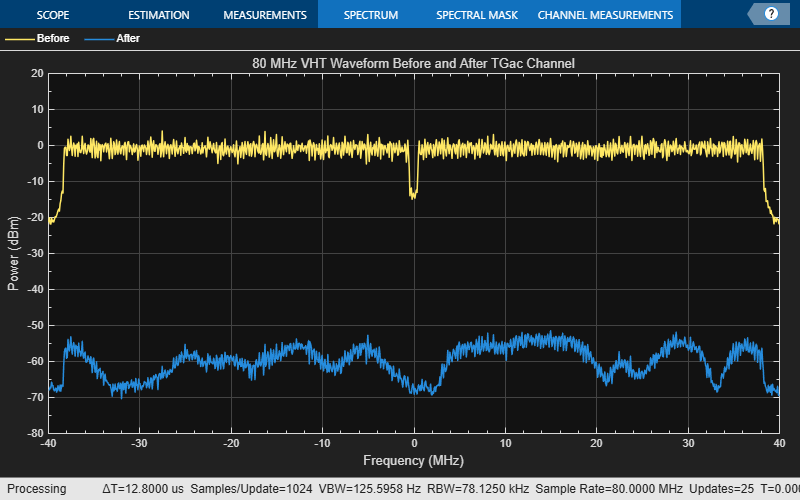Path loss accounts for the roughly 50 to 60 dB of separation between the waveform before and after it passes through the TGac channel. The path loss results from the default transmitter-to-receiver distance of 3 meters, and from shadowing effects. The signal level variation shows the frequency selectivity of the delay profile across the frequency spectrum.

### Pass HT Waveform Through TGn SISO Channel

Create a bit stream to use when generating the WLAN HT format waveform.

```bits = randi([0 1],1000,1); ```

Create an HT configuration object, and generate an HT waveform.

```ht = wlanHTConfig; preChHT = wlanWaveformGenerator(bits,ht); ```

Pass the signal through a TGn SISO channel with AWGN noise (SNR=10 dB) and a receiver with a 9 dB noise figure. Recall that the channel model sampling frequency is equal to the bandwidth in this example. Set parameters using `Name,Value` pairs.

Create a TGn channel object. Set the channel model sampling frequency and channel bandwidth, enable path loss and shadowing, and use the Model-F delay profile.

```fs = 20e6; % Channel model sampling frequency equals the channel bandwidth tgnChan = wlanTGnChannel('SampleRate',fs,'LargeScaleFadingEffect', ... 'Pathloss and shadowing','DelayProfile','Model-F'); ```

Pass the HT waveform through a TGn channel. Use the `awgn` function to add channel noise at an SNR level of 10 dB.

```postChHT = awgn(tgnChan(preChHT),10,'measured'); ```

Create an `AWGN Channel` object to add receiver noise.

```rxNoise = comm.AWGNChannel('NoiseMethod','Variance', ... 'VarianceSource','Input port'); ```

Pass the HT waveform through the receiver. Choose an appropriate noise variance, nVar, for setting the receiver noise level. Here, the receiver noise is based on the noise variance for a receiver with a 9 dB noise figure. `nVar` = kTBF, where k is Boltzmann's constant, T is the ambient temperature of 290 K, B is the bandwidth, and F is the receiver noise figure.

```nVar = 10^((-228.6 + 10*log10(290) + 10*log10(fs) + 9)/10); rxHT = rxNoise(postChHT, nVar); ```

Display a spectrum analyzer with before-channel and after-channel waveforms. Use `SpectralAverages` = 10 to reduce noise in the plotted signals.

```title = '20 MHz HT Waveform Before and After TGn Channel'; saScope = dsp.SpectrumAnalyzer('SampleRate',fs,'ShowLegend',true,... 'AveragingMethod','Exponential','ForgettingFactor',0.99,'Title',title,... 'ChannelNames',{'Before','After'}); saScope([preChHT,postChHT]) ```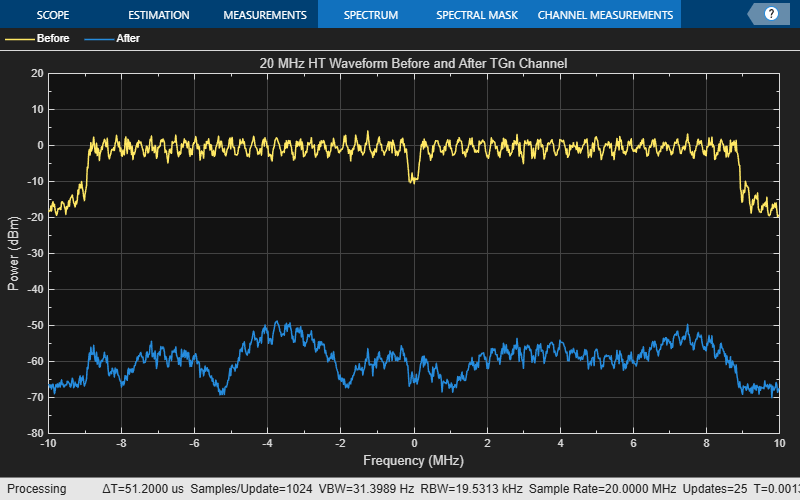Path loss accounts for the roughly 50 to 60 dB of separation between the waveform before and after it passes through the TGn channel. The path loss results from the default transmitter-to-receiver distance of 3 meters, and from shadowing effects. The signal level variation shows the frequency selectivity of the delay profile across the frequency spectrum.

### Pass Non-HT Waveform Through 802.11g Channel

Create a bit stream to use when generating the WLAN Non-HT format waveform.

```bits = randi([0 1],1000,1); ```

Create a non-HT configuration object, and generate a non-HT waveform.

```nht = wlanNonHTConfig; preChNonHT = wlanWaveformGenerator(bits,nht); ```

Calculate free-space path loss for a transmitter-to-receiver separation distance of 3 meters. Create an 802.11g channel object with a 3 Hz maximum Doppler shift and an RMS path delay equal to two times the sample time. Recall that the channel model sampling frequency is equal to the bandwidth in this example. Create an AWGN channel object.

```dist = 3; fc = 2.4e9; pathLoss = 10^(-log10(4*pi*dist*(fc/3e8))); fs = 20e6; % Channel model sampling frequency equals the channel bandwidth maxDoppShift = 3; trms = 2/fs; ch802 = comm.RayleighChannel('SampleRate',fs,'MaximumDopplerShift',maxDoppShift,'PathDelays',trms); ```

Pass the non-HT waveform through an 802.11g channel. Use the `awgn` function to add channel noise at an SNR level of 10 dB.

```postChNonHT = awgn(ch802(preChNonHT),10,'measured'); ```

Create an `AWGN Channel` object to add receiver noise.

```rxNoise = comm.AWGNChannel('NoiseMethod','Variance', ... 'VarianceSource','Input port'); ```

Pass the non-HT waveform through the receiver. Choose an appropriate noise variance, `nVar`, for setting the receiver noise level. Here, the receiver noise is based on the noise variance for a receiver with a 9 dB noise figure. `nVar` = kTBF, where k is Boltzmann's constant, T is the ambient temperature of 290 K, B is the bandwidth, and F is the receiver noise figure.

```nVar = 10^((-228.6 + 10*log10(290) + 10*log10(fs) + 9)/10); rxNonHT = rxNoise(postChNonHT, nVar)* pathLoss; ```

Display a spectrum analyzer with before-channel and after-channel waveforms. Use `SpectralAverages` = 10 to reduce noise in the plotted signals.

```title = '20 MHz Non-HT Waveform Before and After 802.11g Channel'; saScope = dsp.SpectrumAnalyzer('SampleRate',fs,'ShowLegend',true,... 'AveragingMethod','Exponential','ForgettingFactor',0.99,'Title',title,... 'ChannelNames',{'Before','After'}); saScope([preChNonHT,rxNonHT]) ```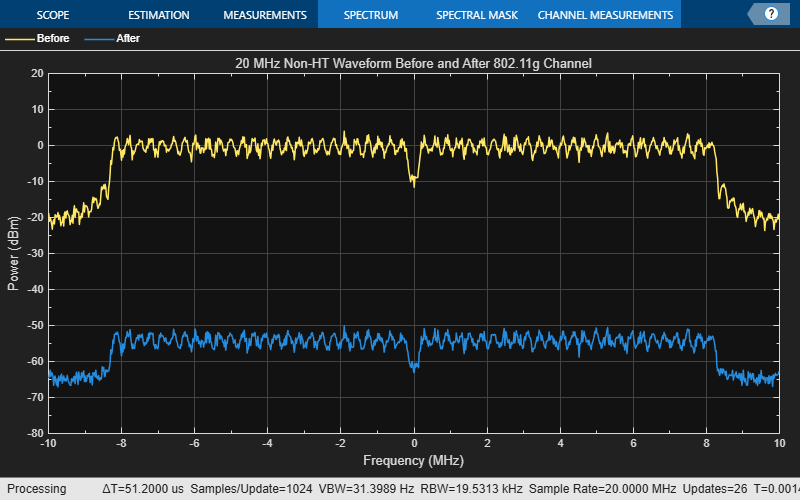Free-space path loss accounts for the roughly 50 to 60 dB of separation between the waveform before and after it passes through the 802.11g channel. The path loss results from the specified transmitter-to-receiver distance of 3 meters, and from shadowing effects. The signal level variation shows the frequency selectivity of the delay profile across the frequency spectrum.

### Pass VHT Waveform Through TGac MIMO Channel

Create a bit stream to use when generating the WLAN VHT format waveform.

```bits = randi([0 1],1000,1); ```

Create a multi-user VHT configuration object, and generate a VHT waveform. Set the number of transmit antennas to four. Set the number of space-time streams and the number of receive antennas to 3. Because the number of transmit antennas is not equal to the number of space-time streams, the spatial mapping is not direct. Set the spatial mapping to Hadamard.

```ntx = 4; nsts = 3; nrx = 3; vht = wlanVHTConfig('NumTransmitAntennas',ntx, ... 'NumSpaceTimeStreams',nsts,'SpatialMapping','Hadamard'); preChVHT = wlanWaveformGenerator(bits,vht); ```

Create TGac MIMO channel and AWGN channel objects. Recall that the channel model sampling frequency is equal to the bandwidth in this example. Disable large-scale fading effects.

```cbw = vht.ChannelBandwidth; fs = 80e6; % Channel model sampling frequency equals the channel bandwidth tgacChan = wlanTGacChannel('SampleRate',fs,'ChannelBandwidth',cbw,... 'NumTransmitAntennas',ntx,'NumReceiveAntennas',nrx); tgacChan.LargeScaleFadingEffect = 'None'; ```

Pass the VHT waveform through a TGac channel. Use the `awgn` function to add channel noise at an SNR level of 10 dB.

```postChVHT = awgn(tgacChan(preChVHT),10,'measured'); ```

Create an `AWGN Channel` object to add receiver noise.

```rxNoise = comm.AWGNChannel('NoiseMethod','Variance', ... 'VarianceSource','Input port'); ```

Pass the multi-user VHT waveform through a noisy TGac channel. Choose an appropriate noise variance, nVar, for setting the AWGN level. Here, the AWGN level is based on the noise variance for a receiver with a 9 dB noise figure. `nVar` = kTBF, where k is Boltzmann's constant, T is the ambient temperature of 290 K, B is the bandwidth, and F is the receiver noise figure.

```nVar = 10^((-228.6 + 10*log10(290) + 10*log10(fs) + 9)/10); rxVHT = rxNoise(postChVHT,nVar); ```

Display a spectrum analyzer showing the multiple streams after the channel effects have been added. Use `SpectralAverages` = 10 to reduce noise in the plotted signals.

```title = '80 MHz VHT 4x3 MIMO Waveform After TGac Channel'; saScope = dsp.SpectrumAnalyzer('SampleRate',fs,'ShowLegend',true,... 'AveragingMethod','Exponential','ForgettingFactor',0.99,'Title',title,... 'ChannelNames',{'RX1','RX2','RX3'}); saScope(rxVHT) ```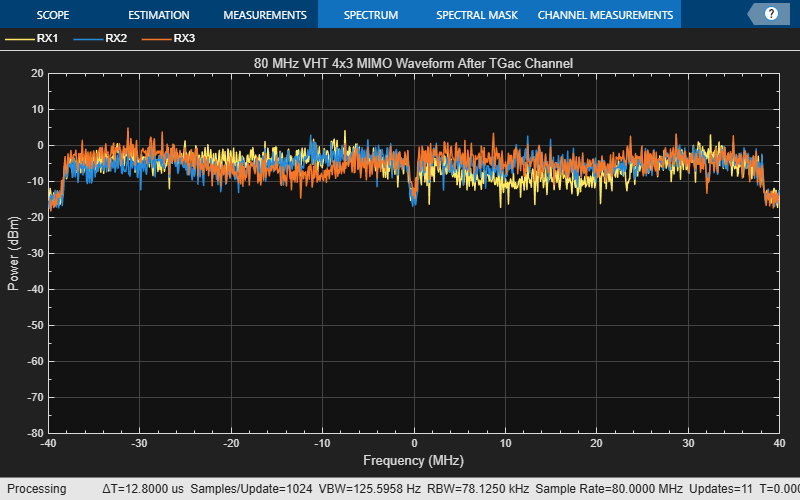The overlaid signals show the TGac channel variation between the received streams.

### References

 Erceg, V., L. Schumacher, P. Kyritsi, et al. TGn Channel Models. Version 4. IEEE 802.11-03/940r4, May 2004.

 Breit, G., H. Sampath, S. Vermani, et al. TGac Channel Model Addendum. Version 12. IEEE 802.11-09/0308r12, March 2010.